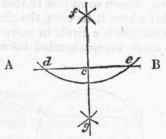## Problem L

To divide a given line A B into two equal parts.

From the end of the line A and B, Fig. 1, as centres, and with any opening of the compasses greater than half A B, describe arches, cutting each other in c and d. Draw the line ed; and the point E, where it cuts A B, will be the middle required. This is often effected by practical men assuming a distance as half, and setting off from each end of the line, and then bisecting the space between the distances set off by the eye.

## Problem II

To raise a perpendicular to a given line A B, from a point given at C.

Case 1. When the given point is near the middle of the line, on each side of the point C, Fig. 2. Take any two equal distances, C d and C e; from d and e, with any radius or opening of the compasses greater than C d or C e, describe two arcs cutting each other inf. Lastly, through the points f C, draw the line f g, and it will be the perpendicular required.

Case 2. When the point is at, or near the end of the line, take any point d, Fig. 3, on the side of the line on which the perpendicular is to be drawn, and with the radius or distance d c, describe the arc e c f, cutting e B in e and c. Through the centre d, and the point e, draw the line e d f, cutting the arc e c f in f. Through the points f c, draw the line f c, and it will be the perpendicular required.

## Problem III

From a given point f, to let fall a perpendicular upon a given line A B.

Case 1. From the point f Fig. 2, with any radius, describe the arc de, cutting A B in e and d. From the points e d, with the same or any other radius, describe two arcs, cutting each other in g. Through the points f and g draw the line f g, and f C will be the perpendicular required.

Case 2. If the point be nearly over the end of the line, from it as at f, Fig. 3, draw a line e f obliquely to the other end; bisect e f in d, and with the radius d e draw the arc f c e, cutting the given line at c, to which the required perpendicular from f is to be drawn.

Fig. 1.Fig. 2.Fig. 3.## Problem IV

To make an angle equal to another angle which is given, as a B b.

From the point B, Fig. 4 with any radius, describe the arc a b, cutting the legs B a,Bb, in the points a and b. Draw the line D b, and from the point D, with the same radius as before, describe the arc b f, cutting D b in b. Take the distance b a, and apply it to the arc b f, from b to f. Lastly, through the points D f, draw the line D f, and the angle b D f will be equal to the angle b B a, as was required.

## Problem V

To divide a given angle, ABC, into two equal parts or angles.

From the point B, Fig. 5, with any radius, describe the arc A C. From A and C, with the same, or any other radius, describe arcs cutting each other in d. Draw the line B d, and it will bisect the angle A B C as was required.